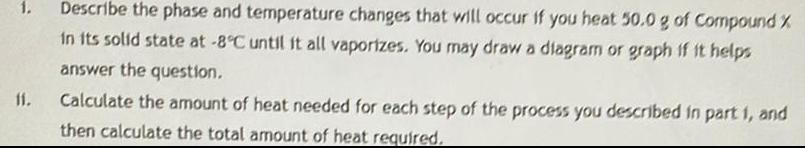Question:

# Describe the phase and temperature changes that will occur

Last updated: 8/8/2022Describe the phase and temperature changes that will occur if you heat 50.0 g of Compound X in its solid state at -8°C until it all vaporizes. You may draw a diagram or graph if it helps answer the question. Calculate the amount of heat needed for each step of the process you described in part 1, and then calculate the total amount of heat required.